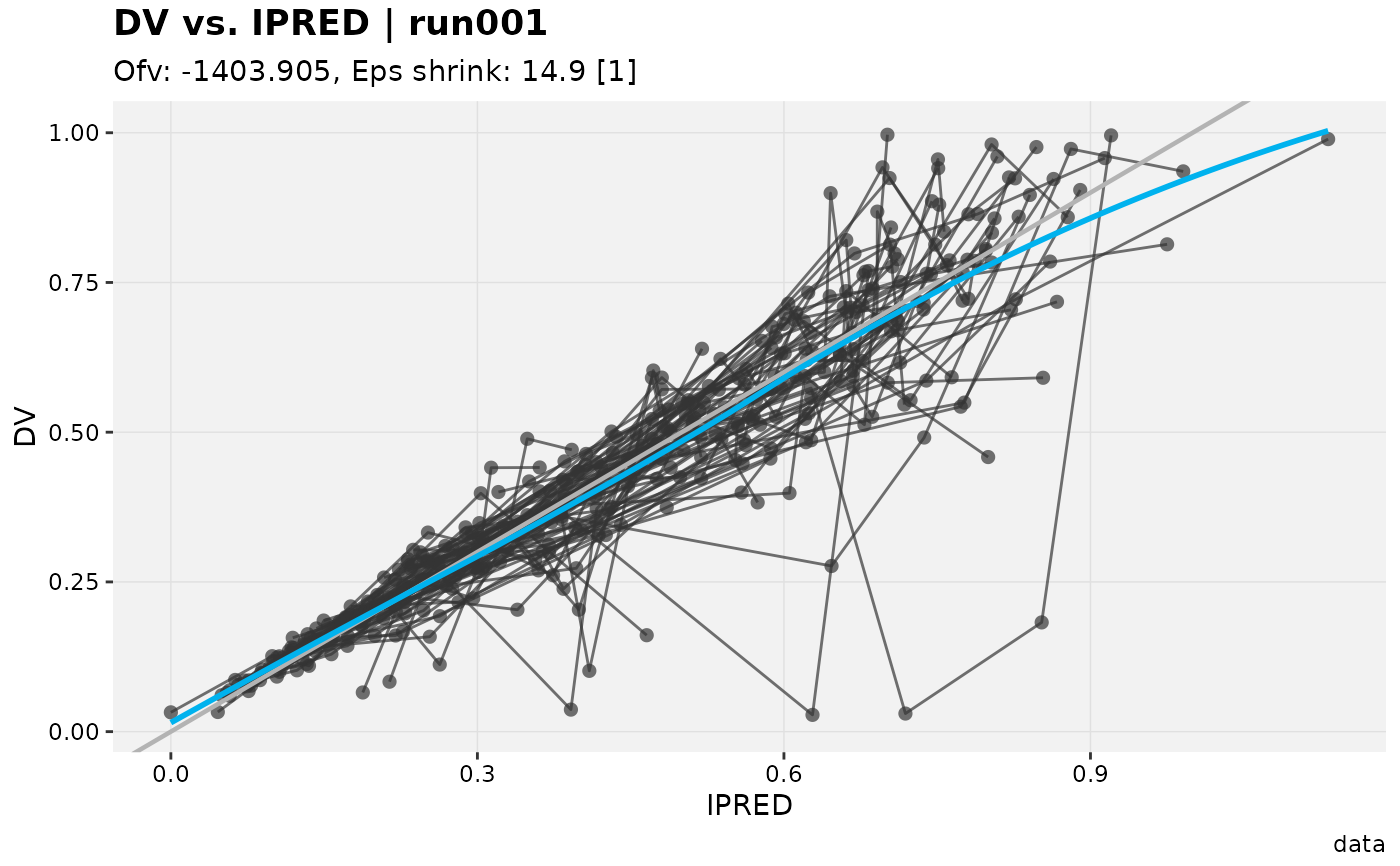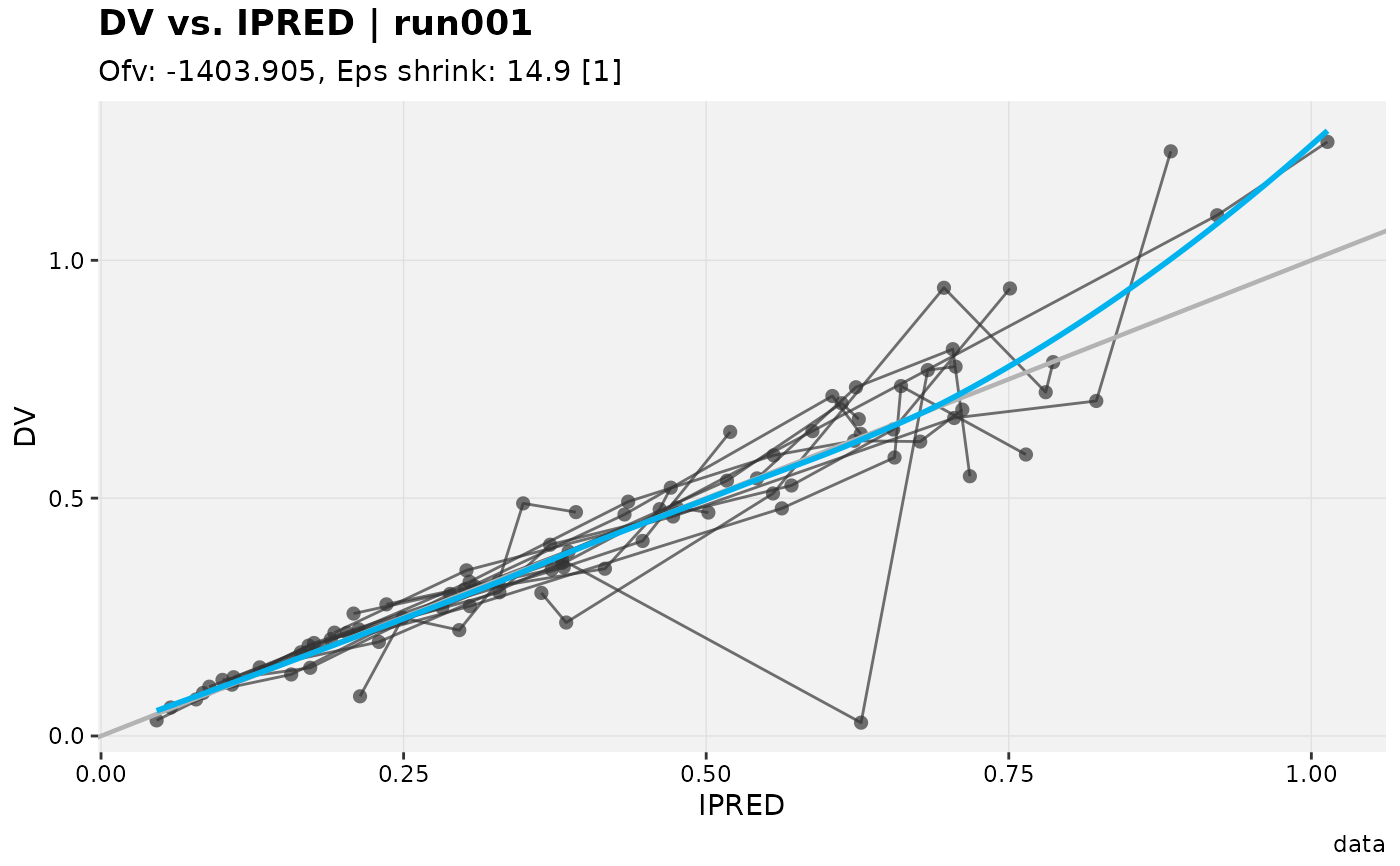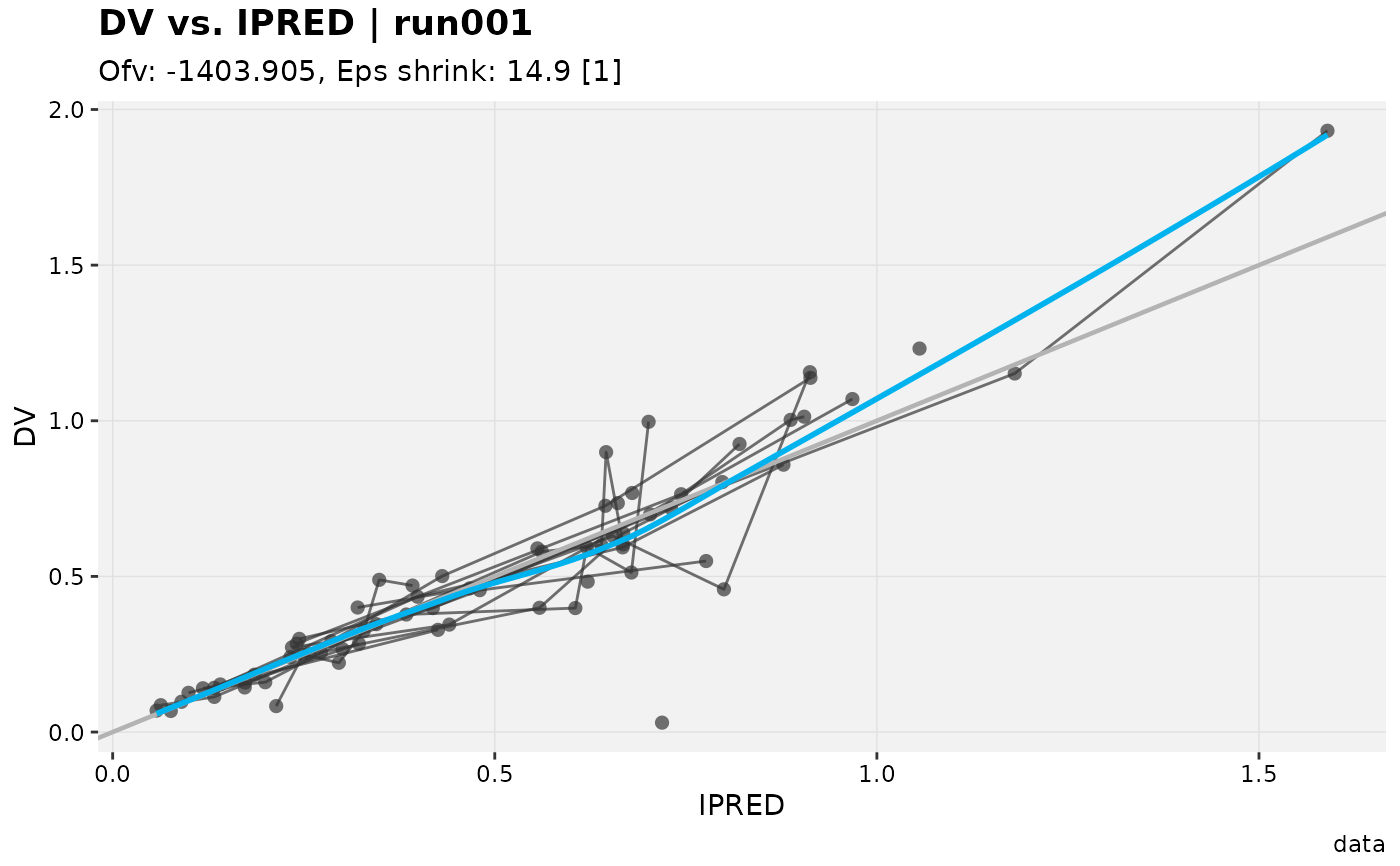Use filter() to select rows/cases where conditions are true. Unlike base subsetting, rows where the condition evaluates to NA are dropped. Use slice() to select row/cases by their position

# S3 method for xpose_data
filter(.data, ..., .problem, .source, .where)

# S3 method for xpose_data
slice(.data, ..., .problem, .source, .where)

# S3 method for xpose_data
distinct(.data, ..., .problem, .source, .where)

## Arguments

.data An xpose database object. Name-value pairs of expressions. Use NULL to drop a variable. These arguments are automatically quoted and evaluated in the context of the data frame. They support unquoting and splicing. See the dplyr vignette("programming") for an introduction to these concepts. The problem from which the data will be modified The source of the data in the xpdb. Can either be 'data' or an output file extension e.g. 'phi'. A vector of element names to be edited in special (e.g. .where = c('vpc_dat', 'aggr_obs') with vpc).

## Examples

# Subset by condition
xpdb_ex_pk %>%
filter(DV < 1, .problem = 1) %>%
dv_vs_ipred()#> Using data from $prob no.1#> Filtering data by EVID == 0#> geom_smooth() using formula 'y ~ x'#> geom_smooth() using formula 'y ~ x'# Subset by positions xpdb_ex_pk %>% slice(1:100, .problem = 1) %>% dv_vs_ipred()#> Using data from$prob no.1#> Filtering data by EVID == 0#> geom_smooth() using formula 'y ~ x'#> geom_smooth() using formula 'y ~ x'# Deduplicate rows
xpdb_ex_pk %>%
distinct(TIME, .problem = 1) %>%
dv_vs_ipred()#> Using data from \$prob no.1#> Filtering data by EVID == 0#> geom_smooth() using formula 'y ~ x'#> geom_smooth() using formula 'y ~ x'